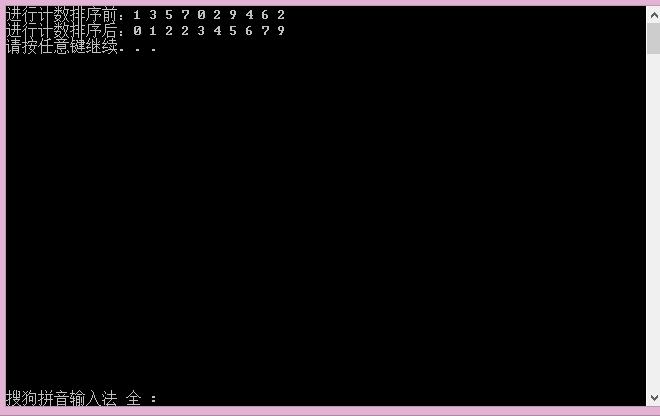【数据结构】中的计数排序(CountSort)

1.计数排序的原理：设被排序的数组为A,排序后存储到B，C为临时数组。所谓计数，首先是通过一个数组C[i]计算大小等于i的元素个数，此过程只需要一次循环遍历就可以；在此基础上，计算小于或者等于i的元素个数，也是一重循环就完成。下一步是关键：逆序循环，从length[A]到1，将A[i]放到B中第C[A[i]]个位置上。原理是：C[A[i]]表示小于等于a[i]的元素个数，正好是A[i]排序后应该在的位置。而且从length[A]到1逆序循环，可以保证相同元素间的相对顺序不变，这也是计数排序稳定性的体现。在数组A有附件属性的时候，稳定性是非常重要的。

2.计数排序的前提及适用范围:A中的元素不能大于k,而且元素要作为数组的下标，所以元素应该为非负整数。而且如果A中有很大的元素，不能够分配足够大的空间。所以计数排序有很大局限性，其主要适用于元素个数多，但是普遍不太大而且总小于k的情况，这种情况下使用计数排序可以获得很高的效率。

#include<iostream>
using namespace std;
#include<assert.h>
#pragma once

void CountSort(int *a, size_t n)
{
assert(a);
int max = a;
int min = a;
for (size_t i = 0; i < n; ++i)
{
if (a[i] > max)
{
max = a[i];
}
if (a[i] < min)
{
min = a[i];
}
}
int range = max - min + 1;
int *Counts = new int[range];
memset(Counts, 0, sizeof(size_t)*range);

for (size_t i = 0; i < n; ++i)
{
Counts[a[i] - min]++;
}
size_t j = 0;

for (size_t i = 0; i < n; ++i)
{
while (Counts[i]--)
{
a[j++] = i + min;
}
}
delete[] Counts;
}
void printArray(int *a, size_t n)
{
for (size_t i = 0; i < n; i++)
{
cout << a[i] << " ";
}
cout << endl;
}
int main()
{
int arr[] = { 1, 3, 5, 7, 0, 2, 9, 4, 6, 2 };
//int arr[] = { 4, 38, 65, 97, 76, 13, 27, 49 };
int n = sizeof(arr) / sizeof(arr);
cout << "进行计数排序前：";
printArray(arr, n);
CountSort(arr, n);
cout << "进行计数排序后：";
printArray(arr, n);
system("pause");
return 0;
}